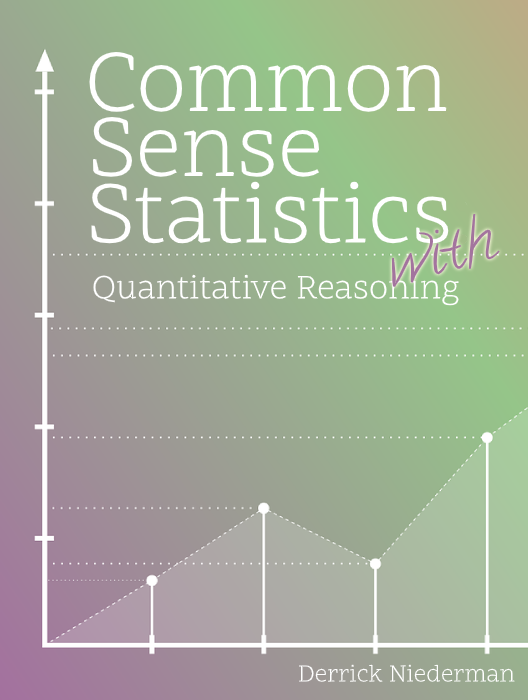# Common Sense Statistics - With Quantitative Reasoning

Finally! An introductory statistics book that’s truly introductory. Common Sense Statistics was written with the understanding that 1) not everyone excelled in math in high school, 2) many students sign up for introductory statistics not because of a lifelong curiosity about the subject but because they have to (horrors!), and 3) if you want to convert these students into satisfied customers, you have to dial down the formalism that has dogged statistics classes for far too long. The goal of Common Sense Statistics is simple: It aspires to be the friendliest math textbook its readers have ever seen. But don’t take my word for it. Have a look inside and see what you think!

### INTRODUCTION AND ACKNOWLEDGMENTS

What's this book all about? Plus a brief bio, if you're interested.

### A FRESH LOOK AT PERCENTAGES

Review material. Basic in theory but sometimes not so basic: Treating percentage changes as multipliers, linking percentage returns using multipliers, how tax brackets really work, and common mistakes with percentages.

### THE LAWS OF AVERAGES

Means and medians. Weighted averages. Quartiles and percentiles. Routine stuff, but with some exercises that aren't exactly routine.

### MEASURING VARIATION

Trying to make the standard deviation understandable. Plus, the Range Rule, Chebyshev's Theorem and an introduction to z-scores.

### HOW TO READ GRAPHS AND CHARTS

We're all lifetime consumers of someone else's charts. It's time to understand good charts and bad ones. Where charts go wrong along both the x- and y-axes. Pareto charts and the 80/20 principle. Benford's Law.

### PROBABILITY BASICS: COUNTING

The multiplication rule for counting. Factorials. The ${}_nC_r$ function. Calculation of poker odds.

### THE BUILDING BLOCKS OF PROBABILITY

The addition rule. The multiplication rule. Complementary and conditional probabilities.

### PROBABILITY DISTRIBUTIONS

Why distributions are even more important than individual probabilities. An emphasis on discrete probability distributions, with a special focus on the binomial distribution.

### THE NORMAL DISTRIBUTION

Areas under the normal curve. The use of z-score area tables. The normal approximation to the binomial distribution.

### THE CENTRAL LIMIT THEOREM

Why the normal distribution shows up everywhere. The CLT for means and proportions. An applet for endless fun. Emphasis on the types of problems that the CLT enables you to solve.

### CONFIDENCE INTERVALS

Introduction of the error term. Interpretation of confidence intervals. Everything you wanted to know about sample sizes.

### HYPOTHESIS TESTING

Understanding the formal steps through which hypotheses are made and tested statistically.

### ELECTION SPECIAL

A scattershot review of U.S. election history through the lens of polling. Polling advances during the past 100 years. Where polls have gone wrong and what steps have been taken to improve them. Why polling is so hard!